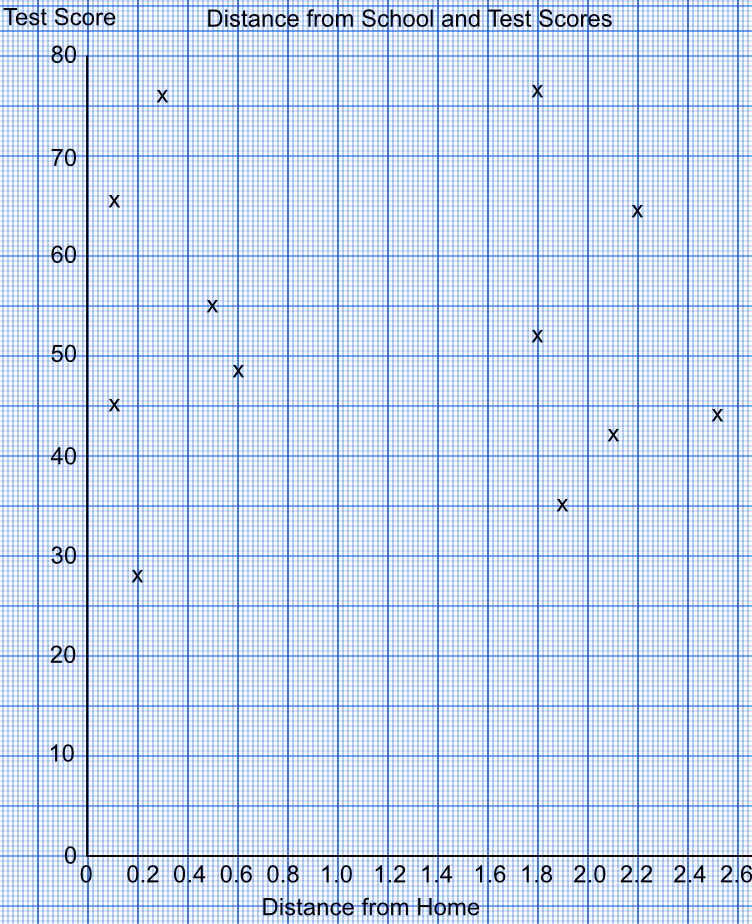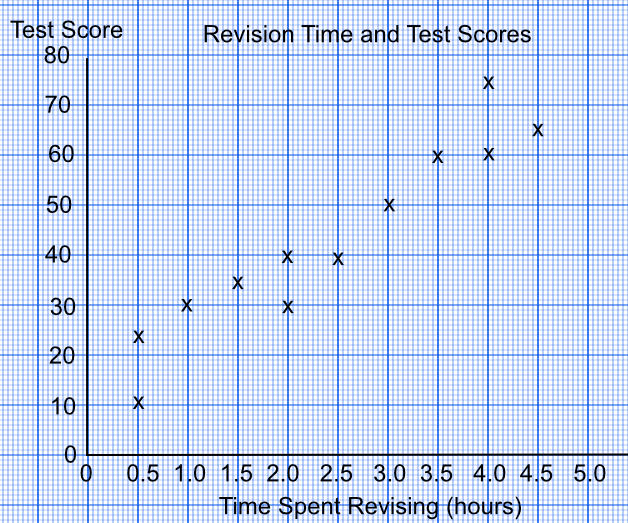Correlation

# Correlation

GCSE(F),

A Scatter Graph shows how two sets of data may be related. Note that, on a scatter graph, the points are not joined up.

The relationship between the two sets of data is known as the Correlation. There are three types of correlation:

If one value increases as the other also increases, then this is a Positive Correlation;

If one value falls as the other increases, then this is a Negative Correlation;

If there is no link between the two sets of data, then there is No CorrelationNote that an increase in one value does not necessarily cause the other value to change: for example, a good result in a Maths test does not cause a good result in a History test.

## Examples

1. A teacher wanted to know whether the performance in a test was linked to the distance the student travelled to school. The teacher obtained the following results:

Distance kmTest Score
0.145
0.165
0.228
0.376
0.555
0.648
1.852
1.876
1.935
2.142
2.264
2.544

By plotting a scatter diagram, or otherwise, indicate the nature of the correlation between the distance the student lives from school, and the results in a test.